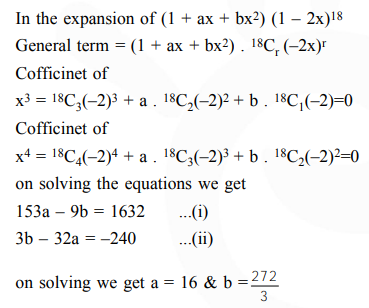# If the coefficients of`
Question:

If the coefficients of $x^{3}$ and $x^{4}$ in the expansion of $\left(1+a x+b x^{2}\right)(1-2 x)^{18}$ in powers of $x$ are both zero, then $(\mathrm{a}, \mathrm{b})$ is equal to :-

1. $\left(16, \frac{251}{3}\right)$

2. $\left(14, \frac{251}{3}\right)$

3. $\left(14, \frac{272}{3}\right)$

4. $\left(16, \frac{272}{3}\right)$

Correct Option: , 4

Solution: